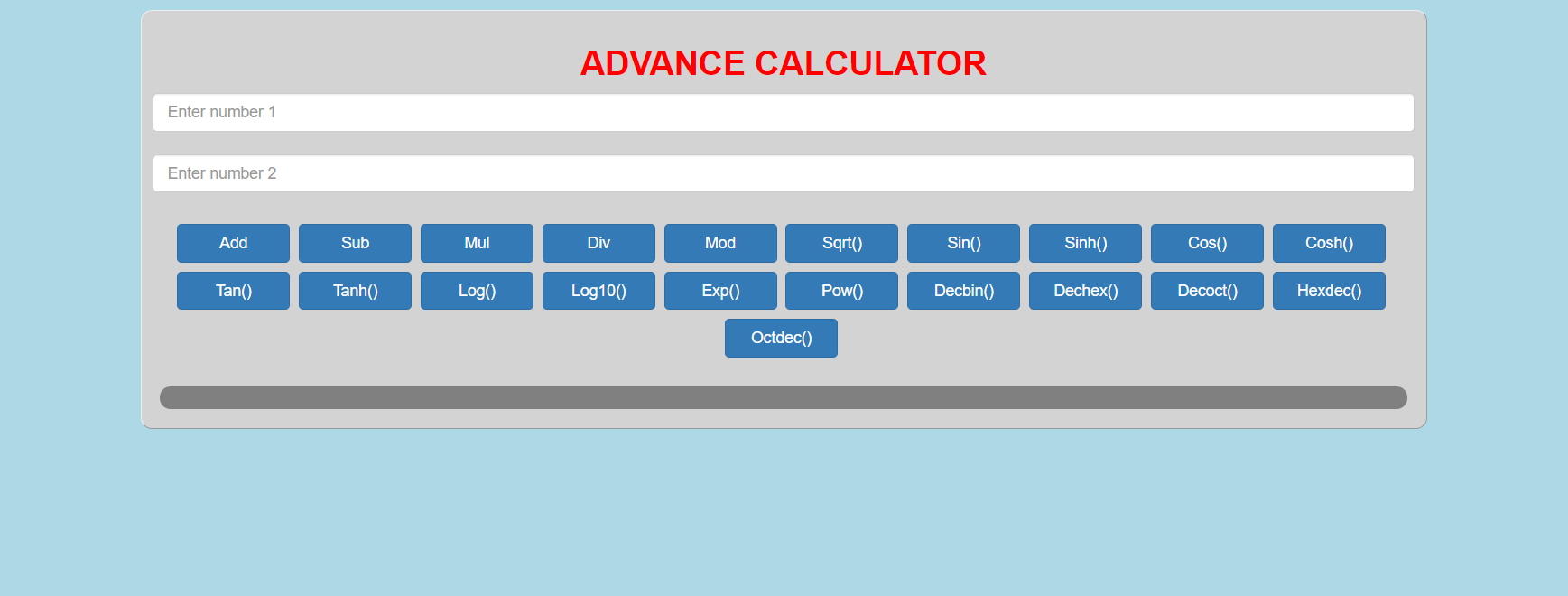# Advance Calculator In PHP With Source Code

Project: Advance Calculator in PHP with source code

The Advance Calculator In PHP is a simple project for solving the mathematical calculations of different numbers. You can see the basic features of the scientific calculator in this project.

The Advance Calculator project is simply in PHP, CSS, and JavaScript. Taking about the features of the Calculator, the user can add, subtract, divide, multiply, check the remainder of any two numbers, find the value of sin, cos, tan, also deal with number conversion from decimal to binary and other numbers. You can just enter the numbers you want to involve in calculations and click the button with the required signs for the result.

This Advance Calculator is in PHP, CSS, JavaScript, and bootstrap. Talking about the features of this system, it’s working function is similar to our scientific calculator functions. The user can perform various calculations. The design of this project is pretty simple so that the user won’t find any difficulties while working on it.

#### How To Run The Project?

To run this project, you must have installed a virtual server i.e XAMPP on your PC (for Windows). This Advance Calculator in PHP with source code is free to download, Use for educational purposes only!

After Starting Apache and MySQL in XAMPP, follow the following steps.

1st Step: Extract file
2nd Step: Copy the main project folder
3rd Step: Paste in xampp/htdocs/

4th Step: Open a browser and go to URL “http://localhost/PHP-mini-project/”